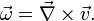## Monday, December 20, 2010

### Vorticity and helicityThere seem to be at least two types of vortex relevant to racing car aerodynamics: transverse vortices and longitudinal vortices. The former spin along axes which lie orthogonal to the direction of travel (as seen in the diagram on the left here), whilst the latter spin along axes parallel to the direction of travel.

Whilst transverse vortices just create drag, a longitudinal vortex can be rather useful, because the centre of such a vortex is a high velocity, low pressure region, which can be used to accelerate or direct airflow in certain directions. The Renault F1 team, for example, used a V-shaped cut in the front wing at Monza this year to create a vortex which accelerated the airflow under the car.

At first sight, there seems to be a particularly simple way to mathematically characterise the distinction between transverse and longitudinal vortices.Given the fluid flow velocity vector field u, the vorticity vector field ω is the curl of the velocity field:Basically, the vorticity vector points along the axis of spin, and the magnitude of the vorticity vector encodes the rate of spin. Given the vorticity vector field, mathematicians introduce several useful additional concepts: vortex lines, vortex sheets and vortex tubes. Technically speaking, vortex lines are the integral curves of the vorticity vector field; this simply means that vortex lines are curves which are tangent to the vorticity field at each point. Vortex sheets, meanwhile, are surfaces which are tangent to the vorticity field at all points. Vortex tubes are three-dimensional regions obtained by taking a 2-dimensional area orthogonal to the vorticity field, and then taking all the vortex lines through that area.

Now, the helicity h is simply defined as the inner product of the velocity and the vorticity:

h = u • ω

Thus, if the streamlines of the fluid are orthogonal to the vorticity, then the helicity is zero. This is the case with a tranverse vortex. In the case of a longitudinal vortex, the helicity is non-zero, and measures how tightly the streamlines corkscrew along a vortex tube. In fact, the helicity of a vortex tube can be defined by integrating the helicity field:It is a theorem of inviscid fluid mechanics that the helicity of a vortex tube is preserved over time. However, if a vortex tube is stretched, (as I presume it must be when it is sucked underneath the floor of a racing car), then its cross-sectional area decreases, and the magnitude of the vorticity ω increases, lowering the pressure at the centre of the vortex.

One might therefore conclude that the stretching of longitudinal vortex tubes should be a principal objective of all racing car aerodynamicists.